# Convert Decimal Hours to Time in Excel

Converting a decimal time value to minutes and hours is easily done in Excel. That is, if the time value is less than 24 hours.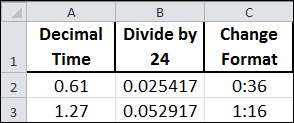## Decimal to Time Conversion

Here are three simple steps to convert decimal to time.

1. Enter a decimal value into a cell
2. Divide by 24
3. Change cell formatting to Time (h:mm)

To change the cell format use keyboard shortcut Ctrl+1 to bring up the Format Cells dialog box, select the Number tab, click Time, then select the h:ss format as shown below.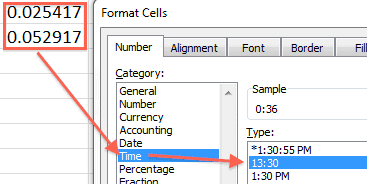If  you have a lot of conversions … Read the rest

# The WEEKDAY Function in Excel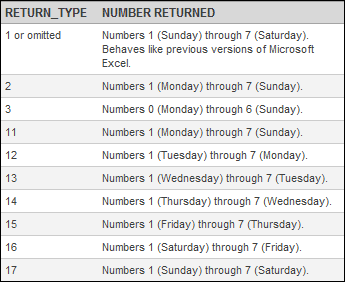Excel 2010 has new return_type arguments for the WEEKDAY Function. How useful they are is debatable, but you never know when you might come across a particular need.

## Excel Weekday Function

The Excel WEEKDAY Function in has two arguments: serial_number and return_type. The serial_number argument is simply a number that represents a date. The return_type argument is optional, and assumed to be 1 if omitted.

Versions prior to Excel 2010 have only the first three return_type arguments: 1,2 3.  The new arguments are 11-17, representing differing combinations for the starting day of the week.… Read the rest

# Date and Time Calculation in Excel

The unit of time in Excel is the Day. By using the NOW Function to show both date and time, you can see the underlying serial number by changing the cell format to General.

This serial number is composed of two parts: the date part is the integer and the time part is the decimal.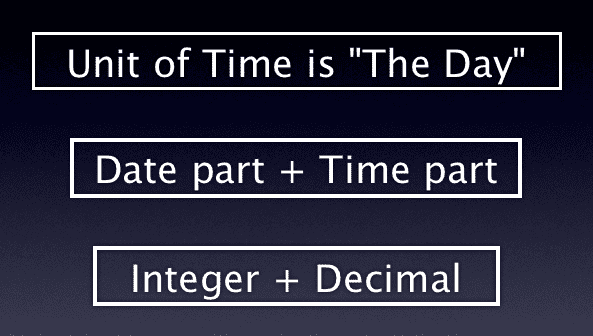The Date serial number is an integer value based upon a Date System, either Windows or Mac. The Time serial number is represented as a decimal fraction because time is considered a portion of a day.

# Convert a Text String Date to Date Serial Number

In previous posts I’ve been going through some formulas designed to extract and convert a text string Date, to a Date serial number.

Given the text string Date below in cell A2, what I normally do is enter a single formula, then change the cell formatting.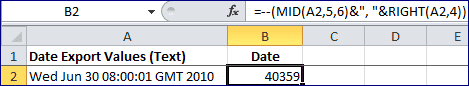The formula above in cell B2 is:

= – –

# The VALUE Function in Excel – Converting Text Dates

The VALUE Function formula in cell G2 has converted the text string Date from cell E2, into a date serial number.

=VALUE(E2)

Until recently, I always used the VALUE Function to do this conversion. It’s something that’s well documented in the Function Arguments.

## Excel Value Function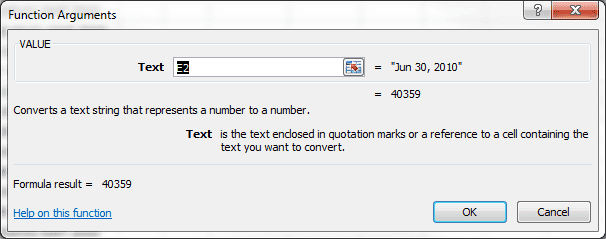## Alternative Formulas to Convert Text Dates

However, all that’s required to change this text string Date into a numeric value (date serial number) is to perform a simple mathematical operation that does not change the value. Excel will then do the conversion for you.

# Extract a Date from Text in Excel

It’s not uncommon for database programs to export data in a text format. I downloaded some data from a web browser, and ended up with Dates that Excel didn’t recognize as Dates, but rather a Text string.

In a previous post I used the Text to Columns feature to extract and convert the Month and Day into a Date Format. Excel used the computer’s System Date to supply the Year. Since all the data happened to be for 2010 there was no problem.

Had there been data from any year other than 2010, … Read the rest

# Generate a List of Dates in Excel

There are times when generating a list of dates comes in handy. Perhaps you need to log something every day for an entire year.

Or maybe you’re tracking stock prices and want to skip the weekend and only use weekday dates. How about a list of dates for each week of the year? Or just month ending days?

Dates for all of these examples can be created with Excel by filling a series.

Here is a bullet list of steps to follow.

• Enter a starting date into a cell
• Right click the cell’s fill handle,